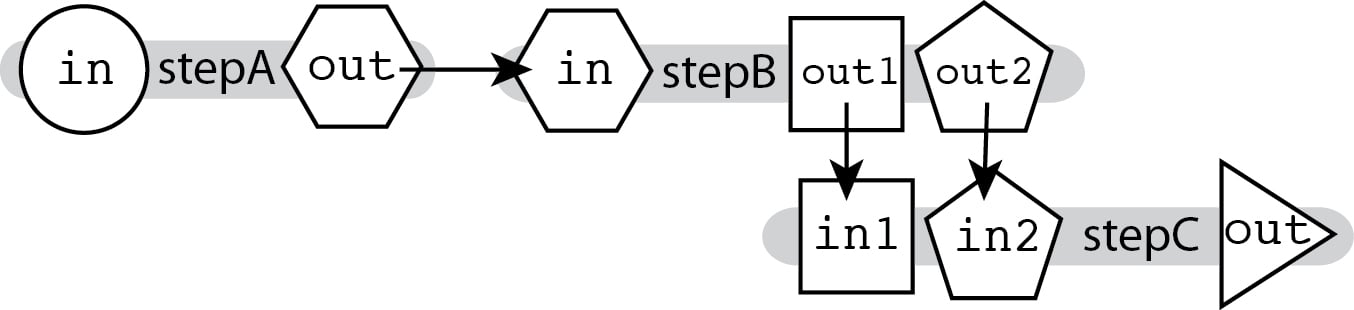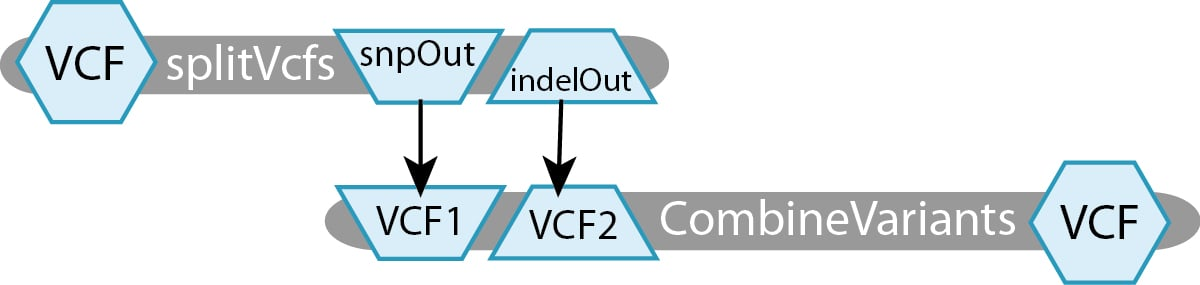# Multi-input / Multi-outputKate Herman
• Updated

WDL articles now live in the openwdl/wdl-docs GitHub repository. Find the updated documentation on the wdl-docs website.

The ability to connect outputs to inputs described in Linear Chaining, which relies on hierarchical naming, allows you to chain together tools that produce multiple outputs and accept multiple inputs, and specify exactly which output feeds into which input.As the outputs for `stepB` are named differently, we can specify where exactly each output goes in the next step's input fields.

```call stepC { input: in1=stepB.out1, in2=stepB.out2 }
```

#### Generic example script

In context, this sort of plumbing would look as follows:

```workflow MultiOutMultiIn {

File firstInput

call stepA { input: in=firstInput }
call stepB { input: in=stepA.out }
call stepC { input: in1=stepB.out1, in2=stepB.out2 }
}

File in

command { programA I=\${in} O=outputA.ext }
output { File out = "outputA.ext" }
}

File in

command { programB I=\${in} O1=outputB1.ext O2=outputB2.ext }
output {
File out1 = "outputB1.ext"
File out2 = "outputB2.ext" }
}

File in1
File in2

command { programB I1=\${in1} I2=\${in2} O=outputC.ext }
output { File out = "outputC.ext" }
}
```

### Concrete example

This workflow uses Picard’s splitVcfs tool to separate the original VCF into two VCF files, one containing only SNPs and the other containing only Indels. The next step takes those two separated VCFs and recombines them into one.For this toy example, we have defined two tasks:

• splitVcfs takes in a `File VCF` and outputs a `File snpOut` and a `File indelOut`.
• CombineVariants takes in a `File VCF1` and a `File VCF2`, producing a `File VCF`.

#### Concrete example script

The workflow described above, in its entirety, would look like this:

```workflow MultiOutMultiInExample {

File inputVCF

call splitVcfs { input: VCF=inputVCF }
call CombineVariants { input: VCF1=splitVcfs.indelOut, VCF2=splitVcfs.snpOut }
}

File VCF

command {
java -jar picard.jar SplitVcfs \
I=\${VCF} \
SNP_OUTPUT=snp.vcf \
INDEL_OUTPUT=indel.vcf
}
output {
File snpOut = "snp.vcf"
File indelOut = "indel.vcf"
}
}

File VCF1
File VCF2

command {
java -jar GenomeAnalysisTK.jar \
-T CombineVariants
-R reference.fasta \
-V \${VCF1} \
-V \${VCF2} \
--genotypemergeoption UNSORTED \
-o combined.vcf
}
output {
File VCF = "combined.vcf"
}
}
```

Note that here for simplicity we omitted the handling of index files, which has to be done explicitly in WDL. For examples of how to do that, see the Tutorials.